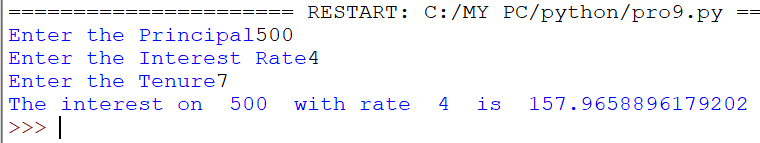# O Level Exam

#### Programming Example

WAP to calculate compound simple interest after taking the principle, rate and time.

`Solution`

```p=int(input("Enter the Principal"))
r=int(input("Enter the Interest Rate"))
t=int(input("Enter the Tenure"))
temp=1+r/100
f=1
for i in range(1,t+1):
f=f*temp
Amount=p*f
interest=Amount-p
print("The interest on ",p," with rate ",r," is ",interest)```
`Output`